## 3.3 Transformations

Transformation of coordinates from one two-dimensional coordinate system to another can be specified by means of a 3x3 transformation matrix. The matrix specifies how a point in one coordinate system is transformed into the corresponding point in another coordinate system.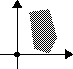The subsequent definitions of the geometric transformations are illustrated with the example polygon in the margin.

A transformation matrix(TM) specifies a transformation of point (x,y) to point (x', y') in the following way: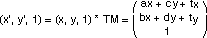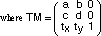The common transformations: scaling, moving (translation), and rotation can easily be described by transformation matrices.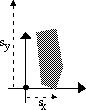Scaling by factor sx in the x dimension and sy in the y dimension is accomplished by: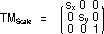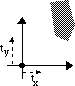Moving (translation) by a specified displacement (tx,ty) is obtained by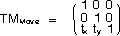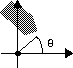Rotating counterclockwise, around the origin of the coordinate system, by an angleis described by the following matrix: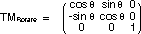The most powerful feature of the matrix application, is that composition of geometric transformations can be expressed as multiplications of the corresponding matrices. That is, a combination of a rotate, move, and scale transformation can be combined into one matrix, and thus reduce the time of calculation of a complex transformation: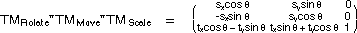The user must be aware of the order of transformations, since matrix multiplication is not commutative. For a more thorough explanation of matrix operations and transformations, see e.g. [Newman 81].

 The Bifrost Graphics System - Reference Manual © 1991-2004 Mjølner Informatics [Modified: Tuesday October 24th 2000 at 15:02]